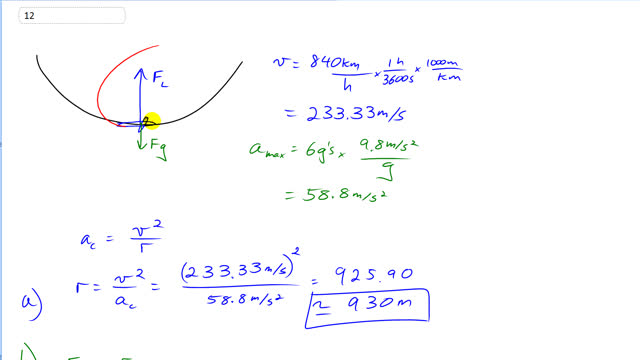## You are here

Question:

A jet pilot takes his aircraft in a vertical loop (Fig. 5–38).

1. If the jet is moving at a speed of 840 km/h at the lowest point of the loop, determine the minimum radius of the circle so that the centripetal acceleration at the lowest point does not exceed 6.0 g’s.
2. Calculate the 78-kg pilot’s effective weight (the force with which the seat pushes up on him) at the bottom of the circle, and
3. at the top of the circle (assume the same speed).Figure 5-38.

Giancoli, Douglas C., Physics: Principles with Applications, 7th Ed., ©2014. Reprinted by permission of Pearson Education Inc., New York.
The question will be visible after logging in, as required by Pearson Education Inc.

1. $930\textrm{ m}$
2. $5400\textrm{ N}$
3. $3800\textrm{ N}$

# Giancoli 7th Edition, Chapter 5, Problem 12

(4:49)